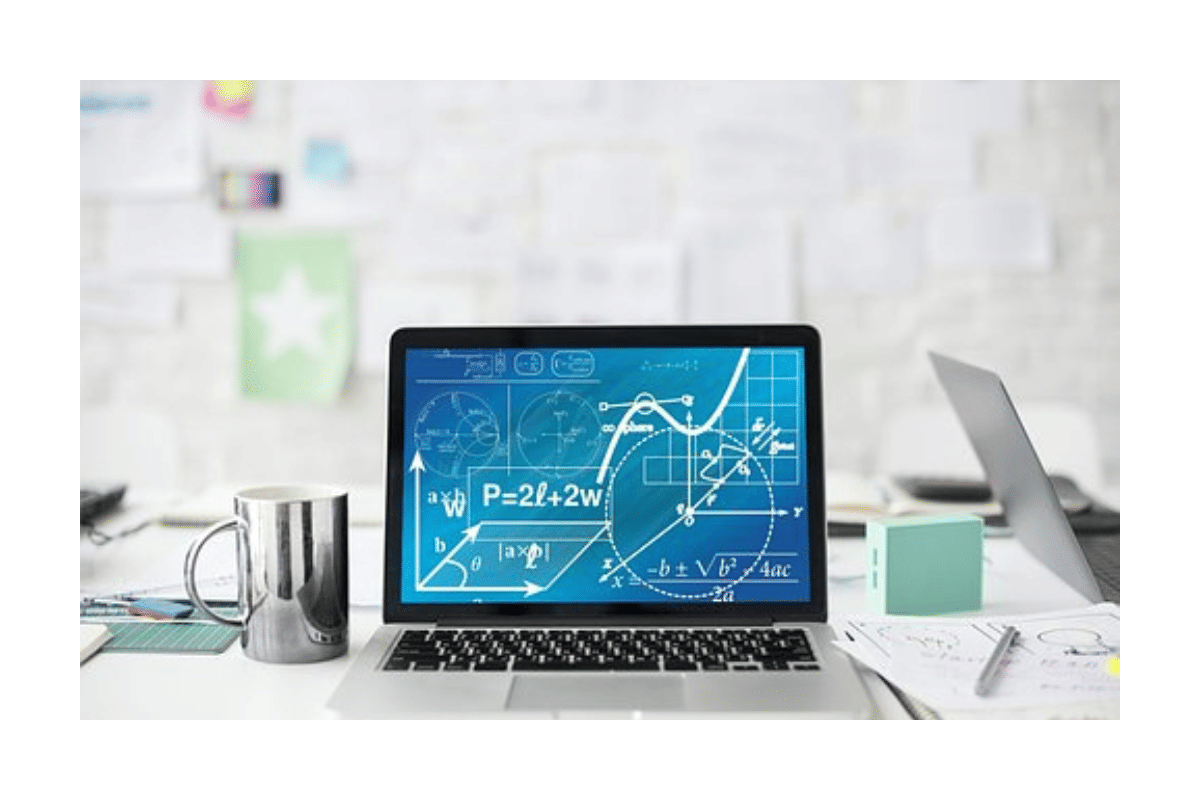# JEE Main 2024 Daily Practice Questions for 2 September 2023

Go through the JEE Main 2024 Practice Questions for Physics, Chemistry, and Mathematics here. Candidates should practice those questions on a regular basis to improve their performance level.JEE Main 2024 Daily Practice Questions for 2 September 2023

JEE Main 2024 Daily Practice Questions: The candidates, who are planning to appear for the JEE Main 2024 exam, should start preparation from now onwards. JEE Main is one of the vital entrance exams, which needs a lot of practice dedicatedly. Hence, the candidates should start daily practice questions to solve on a regular basis. It will help the students to boost their preparation level. It can be assumed that the JEE Main 2024 exam will be held tentatively in January 2024. This means that the candidates are left with only 4 months before the exam, therefore, regular practice from now onwards can uplift their performance level.

## JEE Main 2024 Daily Practice Questions

Check out the JEE Main 2024 daily practice questions on Physics, Chemistry, and Mathematics for 2 September 2023 here:

### Physics:

Ques. A has a half-life of 5 years. Find the fraction of A that might have decayed after 15 years.

1. 1/8

2. 7/8

3. 1/4

4. 3/4

Ques. A particle of mass 10 kg experiences a force F= 5x along x axis. Find work done by force F on the particle from X= 2 to X = 4

1. 10J

2. 15J

3. 30J

4. 40J

Ques. The minimum angle of deviation for the equilateral prism of refractive index n= √2 will be:

1. 60°

2. 40°

3. 30°

4. 15°

### Chemistry:

Ques. What is the Ratio of silica of alumina in cement

1. 3

2. 2

3. 4.5

4. 1.5

Ques. 12g of non-electrolyte A dissolved in 1000 ml of solution. It is isotonic with a 0.05 molar glucose solution. Find the molar mass of A.

Ques. In which of the following options does the species change from paramagnetic to diamagnetic and bond order increase?

### Mathematics:

Ques. The number of seven-digit numbers formed using 1, 2, 3, 4 whose sum of digits is 12, is-

1. 402

2. 413

3. 421

4. 409

Ques. Let the probability of getting a head be thrice of probability of getting tail. A coin is tossed until one head or 3 tails are contained. If the number of trials is 24 then the mean of the experiment is:

1. 28.5

2. 31.5

3. 25

4. 26.5

Ques. Number of 7-digit numbers which contain only digits 1, 2,  and 4, and the sum of the digits of the number is always 12, is:

1. 420

2. 413

3. 252

4. 392

Stay tuned to CollegeDekho for more Education News pertaining to entrance exams and admission. You can also write to us at our E-Mail ID news@collegedekho.com.

/news/jee-main-2024-daily-practice-questions-for-2-september-2023-44679/

### Do you have a question? Ask us.

• Typical response between 24-48 hours

• Get personalized response

• Free of Cost